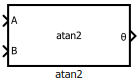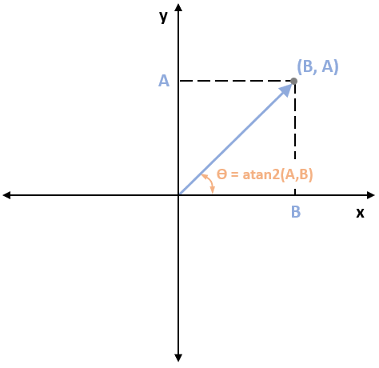# atan2

Since R2020a

•Libraries:
Motor Control Blockset / Controls / Math Transforms
Motor Control Blockset HDL Support / Controls / Math Transforms

## Description

The atan2 block performs the four-quadrant arctangent on two real numbers.

### Equations

This equation describes how the block computes the four-quadrant arctangent (`θ`).

where:

## Ports

### Input

expand all

Real number on the y-axis that you provide as input to the block.

Data Types: `single` | `double` | `fixed point`

Real number on the x-axis that you provide as input to the block.

Data Types: `single` | `double` | `fixed point`

### Output

expand all

Angle represented by arctangent. This is the angle made by a vector from the origin to a specified point (x,y) with the positive x-axis.

Data Types: `single` | `double` | `fixed point`

The following figure shows the representation of input values A, B, and arctangent on the x-y coordinate plane.## Parameters

expand all

Type of approximation that the block uses for atan2 computation:

• `None` — The block does not use any approximation.

• `CORDIC` — The block uses the approximation based on coordinate rotation digital computer (CORDIC) algorithm.

• `Lookup` — The block uses the lookup table based approximation.

Note

When you use the block for code generation:

• `None` option works better with single data type.

• `CORDIC` option works better for FPGA deployment.

• `Lookup` option works better with fixed-point data type.

Unit of the output values.

## Version History

Introduced in R2020a